# Part 7: Year 9 Trigonometry and Its Practical Applications | Free Worksheet

In this article, we introduce trigonometry and discuss some of its practical applications.## Year 9 Trigonometry and its practical applications

Trigonometry is a critical part of what you will learn in the senior years of Maths at high school. Year 9 trigonometry and its practical applications will give you a thorough grounding in what trigonometry is for and how it works.

## NSW Syllabus Outcomes

• Application of trigonometry to solve problems, including problems involving bearings:
• Apply trigonometry to solve right-angled triangle problems.
1. Use a calculator to find values of trigonometric ratios, given angles measured in degrees and minutes, and vice versa.
2. Find length of unknown side given measured angle and vice versa.
• Solve right-angles triangle problems, including those involving direction and angles of elevation and depression.
1. Solve a variety of practical problems involving angles of elevation and depression, including problems for which a diagram is not provided.
1. Draw diagrams to assist in solving practical problems involving angles of elevation and depression (Communicating, Problem Solving).
2. Interpret three-figure bearings and compass bearings.
1. Interpret directions given as bearings and represent them in diagrammatic form (Communicating, Reasoning).
3. Solve a variety of practical problems involving bearings, including problems for which a diagram is not provided.
1. Draw diagrams to assist in solving practical problems involving bearings (Communicating, Problem Solving).
2. Check the reasonableness of solutions to problems involving bearings (Problem Solving).

This may seem overwhelming, but these Syllabus Outcomes are merely explaining why we use Trigonometry.

## Why Trigonometry?

What is Trigonometry? Trigonometry is a word that has its etymological roots in Ancient Greek.

What does this mean? Trigon comes from “triangle”, -metry is a suffix that indicates that the word is a “measurement of”. So, we’re just studying triangles! And triangles are simply shapes with 3 sides and 3 angles.

This means we can use trigonometry to calculate lengths and angles of a triangle! We can then apply this to real life problems.

For example, a captain of a ship would want to know how far away he or she is away from land, to figure this out they would use trigonometry. Similarly, the captain can use it to navigate and follow a course. We’ll look at this when we deal with bearings later in this article.

## Want to test your knowledge of Trigonometry?• The basics of trigonometry
• How to use your calculator to calculate trigonometric ratios
• How to find unknown sides and angles
• The concept of exact ratios will be revised
• Solving practical applications of trigonometry including
• Word problems
• Angles of elevation
• Depression and bearings.

## Assumed Knowledge

To succeed in Year 9 trigonometry:

• Students should be familiar with Pythagoras’ Theorem and simple arithmetic.
• A basic understanding of compass directions is required.

## Year 9 trigonometry and its applications

Trigonometry is a part of mathematics that studies the relationships between a triangle’s angles and its side lengths. These relationships are defined as trigonometric ratios.

Recall Pythagoras’ theorem. It states that the square of the hypothenuse is equal to the sum of the squares of the two other sides of the right-angled triangle.

This is written as:

$$c^2 = a^2 + b^2$$

Remember that the naming convention for a right-angled triangle is with respect to an acute angle (in degrees) inside the triangle.

This is shown below: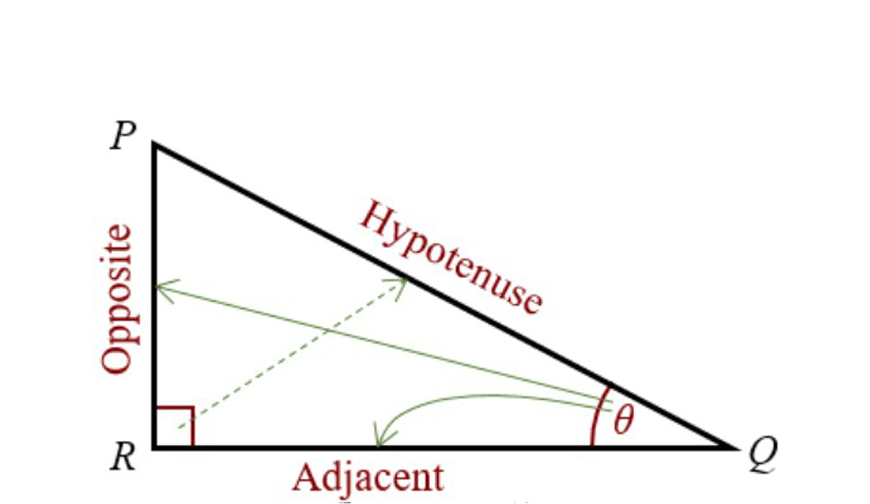Looking at the triangle above, we can write down the three trigonometric ratios:

$$sin⁡θ=\frac{\text{Opposite}}{\text{Hypotenuse}}=\frac{O}{H} \\ cos⁡θ=\frac{\text{Adjacent}}{\text{Hypotenuse}}=\frac{A}{H} \\ tan⁡θ=\frac{\text{Opposite}}{\text{Adjacent}}=\frac{O}{A} \\$$

One way of remembering these ratios is:

Some Old Horse

Caught Another Horse

Taking Oats Away!

## Given one ratio, finding the other ratio

In these types of questions, you’re given one trigonometric ratio and asked to find the other two.

To do this, you need to firstly draw out the right-angled triangle to represent the given trigonometric ratio.

Next, we can use Pythagoras’ theorem to find the missing side and then read off the triangle the other two ratios.

### Example:

1. Given $$sin⁡θ=\frac{3}{5}$$ find $$cos⁡θ$$ and $$tanθ$$.

### Solution:

First we draw the right-angled triangle for the given ratio, $$sin⁡θ=\frac{3}{5}$$.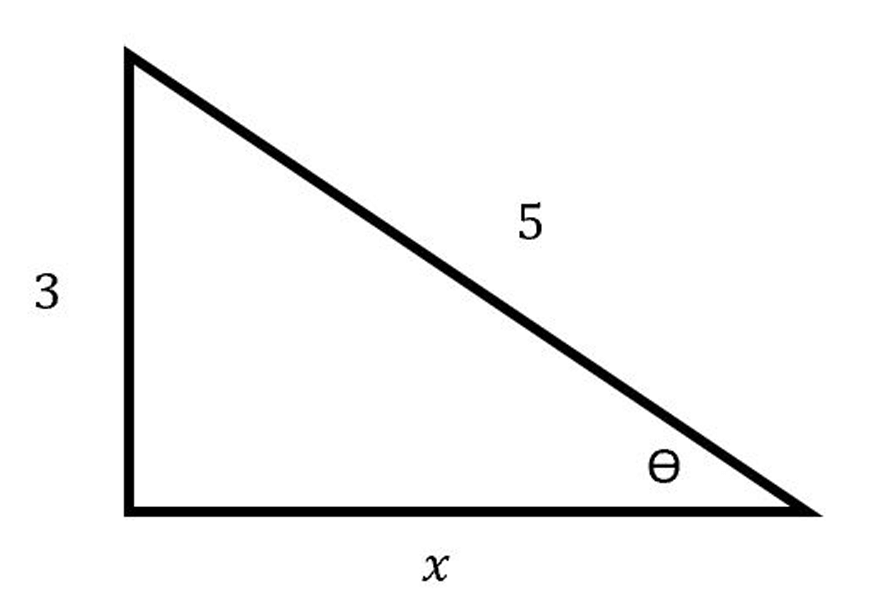Using Pythagoras’ theorem, we can find the unknown side $$x$$.

$$5^2=3^2+x^2 \\ x^2=5^2-3^2 \\ x^2=16 \\ x=4 \\$$

We now have enough information to find the other two ratios.

$$cos⁡θ=\frac{4}{5} \\ tan⁡θ=\frac{3}{4} \\$$

To find the trigonometric ratio for a given angle, we can use the calculator set in degrees mode.

In general, we do:

 $$(sin⁡ \ \text{or} \ cos ⁡\ \text{or} \ tan ) + \text{angle using} (°'”) \ + \ ) \ + =$$

If you know the value of the ratio and want to find the angle, you can use:

 $$\text{SHIFT}+(sin⁡ \ \text{or} \ cos⁡ \ \text{or} \ tan) + \text{given ratio} + \ ) + \ =$$

This will give you the angle in degrees.

## Finding unknown sides of a right-angled triangle

In any right-angled triangle, if we are given an angle and a side, then the other lengths can be found.

To solve these types of questions, we must determine the trigonometric ratio which contains the given angle and side as well as the side we are trying to find.

We can then rearrange the equation to find the value of the unknown side.

### Example:

1. Find the value of $$x$$ in the right-angled triangle below.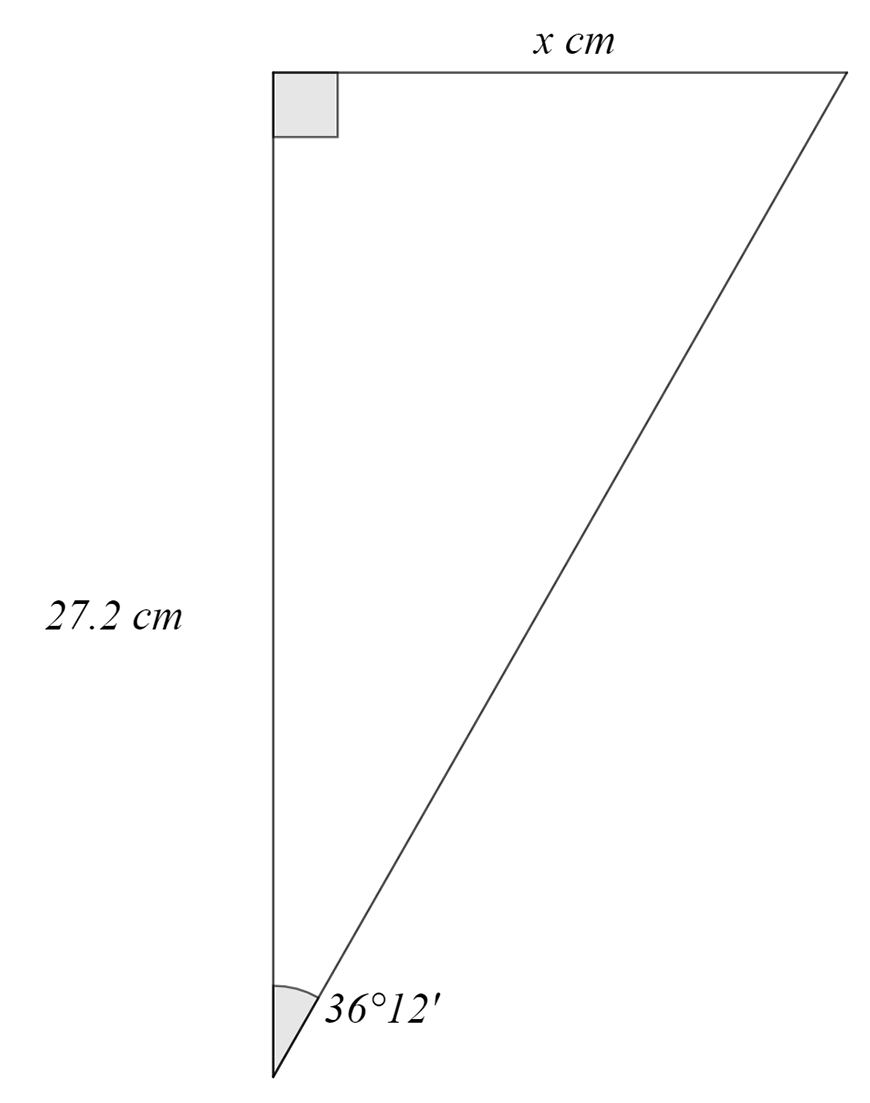### Solution:

From the diagram above we are given the side adjacent (A) to the given angle and must find the opposite (O) side. Hence, we use $$tan⁡θ.$$

$$tan⁡ 36°12′ =\frac{O}{A}=\frac{x}{27.2} \\ ∴x=27.2 tan⁡ 36°12′ =19.91 \\$$

## Finding unknown angles of right-angled triangle

We can find unknown angles in a similar way to finding unknown sides.

If we are given two sides and a mystery angle, we must first determine the trigonometric ratio we should use.

Then we can rearrange and use the calculator to find the angle.

Be careful!

Sometimes you might not be able to find the required angle directly. Instead we can find the other acute angle and then use the angle sum of a triangle is 180 ֯.

### Example

Given the triangle below, find $$β$$.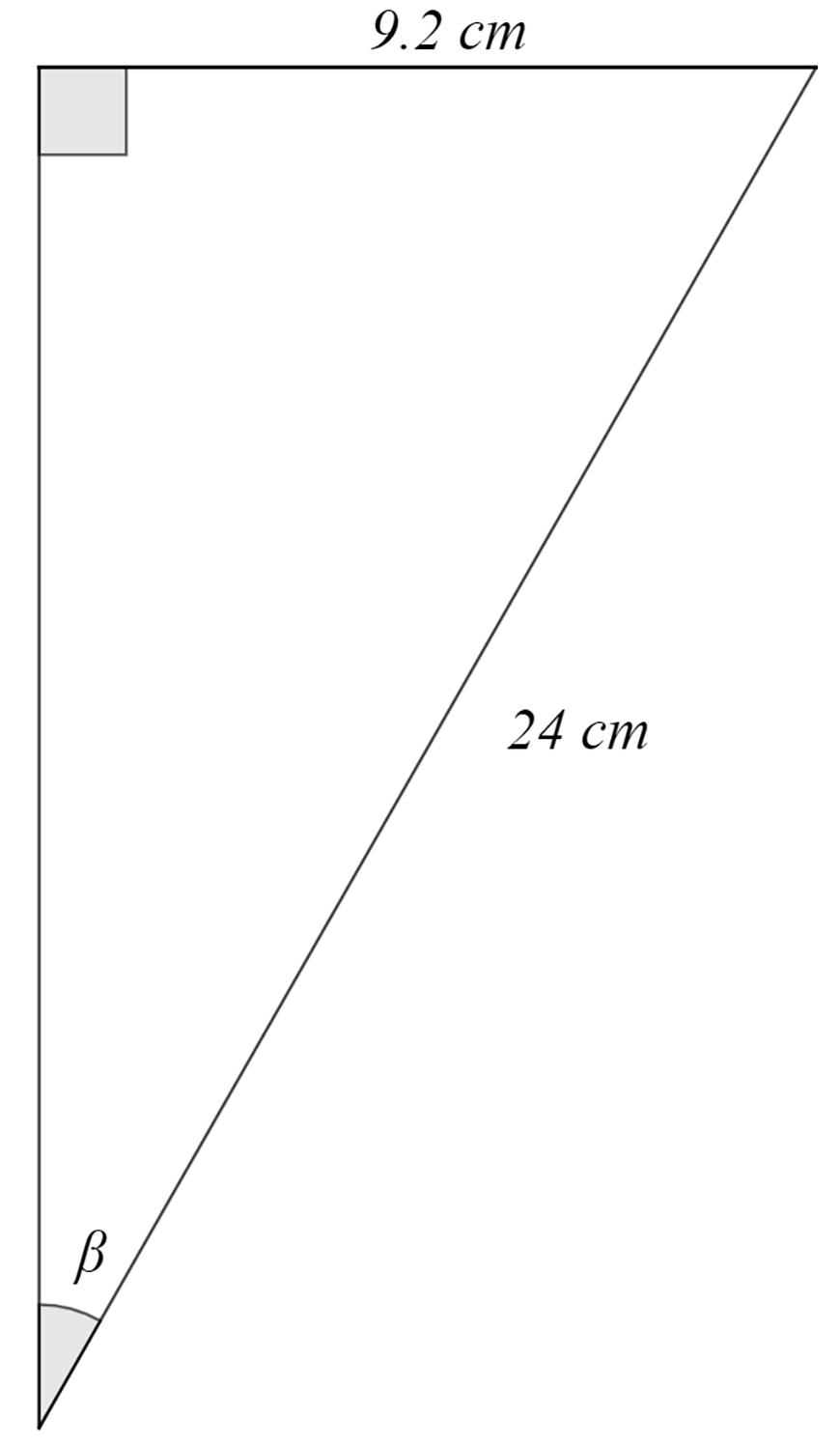### Solution

From the diagram above we can see that we are given the adjacent side (A) and the hypotenuse (H). Therefore, we use the cosine ratio.

$$cosβ=\frac{9.2}{24} \\ β= 67° 28′ \\$$

## Summary of exact ratios

Sometimes you’ll be asked to find the exact solution.

This means we can expect to see the angles $$30°, 45°$$, and $$60°$$. To find the exact solution, we leave any surds as they are… do not evaluate them on the calculator!

It’s easy to memorise all the exact trigonometric ratios by using the following two triangles: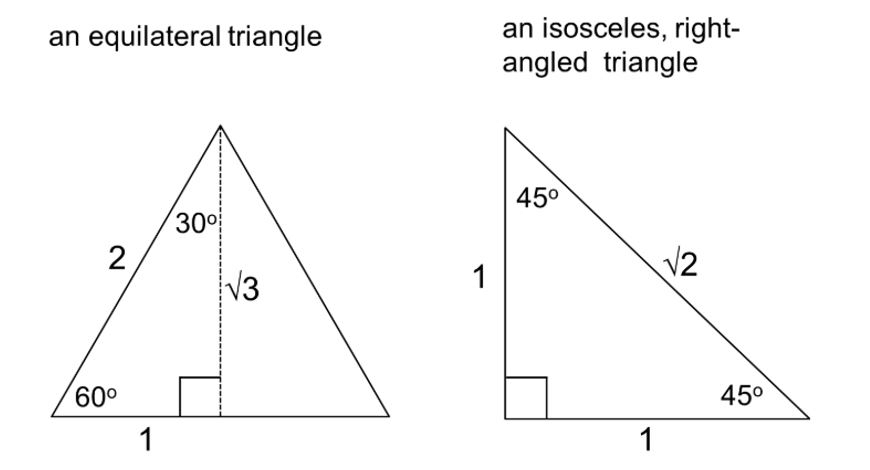Reading off the triangles above, we can create a table of all the exact values.

  $$θ=30°$$ $$θ=45°$$ $$θ=60°$$ $$sin⁡θ$$ $$\frac{1}{2}$$ $$\frac{1}{\sqrt{2}}$$ $$\frac{\sqrt{3}}{2}$$ $$cos⁡θ$$ $$\frac{\sqrt{3}}{2}$$ $$\frac{1}{\sqrt{2}}$$ $$\frac{1}{2}$$ $$tan⁡θ$$ $$\frac{1}{\sqrt{3}}$$ $$1$$ $$\sqrt{3}$$

### Example

Find the exact value of $$x$$ in the triangle below.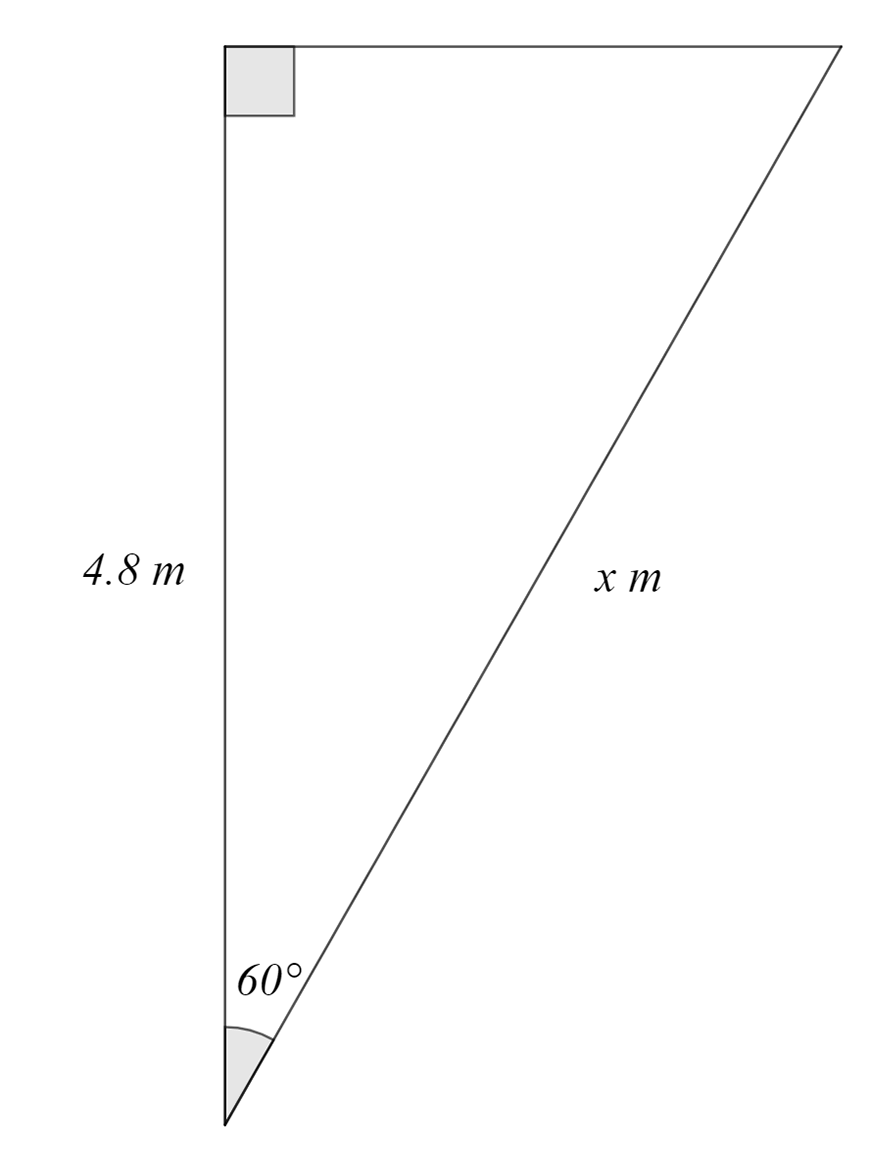### Solution

This is the “find unknown side” type of question.

We can see that we are given an angle and the side adjacent (A) to it, and we wish to find the hypotenuse (H).

Therefore, we use the cosine ratio.

$$cos⁡60°=\frac{4.8}{x} \\ ∴x=\frac{4.8}{cos60°} \\ x=\frac{4.8}{\frac{1}{2}} \\ x=9.6 \\$$

## Angles of elevation and depression

These are the special names given to the angle measured from the horizontal.

If an observer is at A and looks up at C, the angle created is the angle of elevation.

If we consider an observer at looking down at A, this angle is the angle of depression.

Often, you’ll be given word problems involving these two angles.

What we want to do is draw a diagram representing this information and solve by looking at the right-angled triangles formed.

### Example

1. A tower $$XY$$ is 50m tall. From a point $$Z$$ on the ground, the angle of elevation to the top of the tower is $$23°$$. Calculate the distance of $$Z$$ from the base of the tower.

### Solution

First, we draw a diagram of the information given to us. When we look up from $$Z$$ to the top of the tower, $$Y$$, the angle formed is $$23°$$.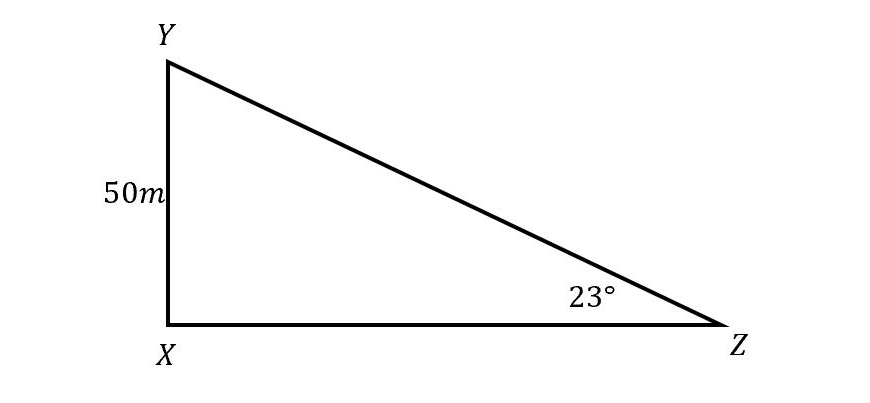We can see that $$XYZ$$ forms a right-angled triangle and we want to find the length $$XZ$$.

We can use the tangent ratio as we have the opposite and adjacent side from the given angle.

$$tan⁡(23°)=\frac{50}{XZ} \\ XZ=\frac{50}{tan⁡23°} \\ XZ=117.8m \\$$

## Bearings

Bearing are angular measurements involved in navigation to indicate directions.

A bearing describes the direction of one location from a given reference point.

There are two ways to measure bearings; compass bearings and true bearings.

Compass bearings start from either North or South and then measure the angle to the point in either the East or West direction.

Whereas, true bearings start at North and use angles between $$0°$$ and $$360°$$  in the clockwise direction to the point.

Be careful!

These types of questions can become quite challenging. You may need to use your knowledge of angles on parallel lines to draw the diagram.

### Example

1. Given the diagram below, write the compass and true bearings of $$P$$ from $$O$$.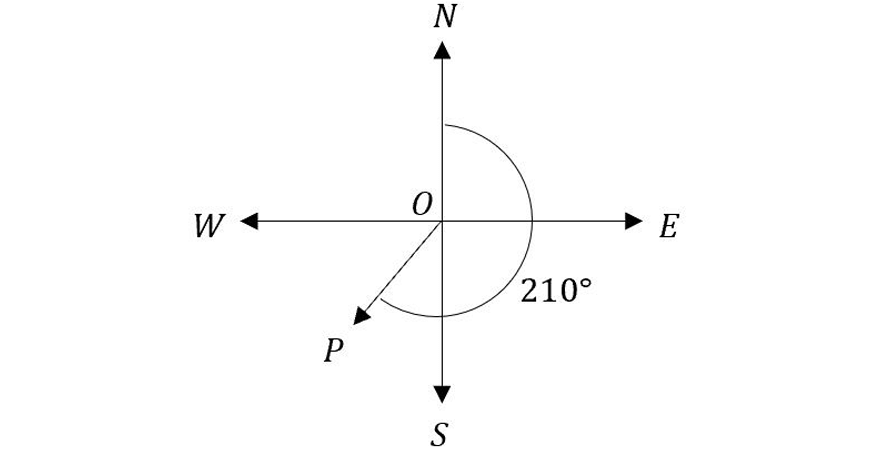### Solution

For compass bearing, point $$P$$ is closer to South than North, so we start with $$S$$. Next, we calculate the angle to $$P$$ from $$S$$.

$$\text{angle}=210°-180°=30°$$

Since $$P$$ is in the West direction, we have,

$$\text{compass bearing}=S30°W$$

For true bearings, we are already given the angle from North, so we can write,

$$\text{true bearing}=210°T$$

## Sample questions

1. If $$sin⁡A=\frac{3}{11}$$ and $$cos⁡B=\frac{5}{9}$$, find $$cos⁡A$$ and $$tan⁡B$$.

2. Use $$∆XYZ$$ to find the length of $$YZ$$ (correct to the nearest mm). Hence find the value of $$x$$ (to the nearest mm) in the diagram below.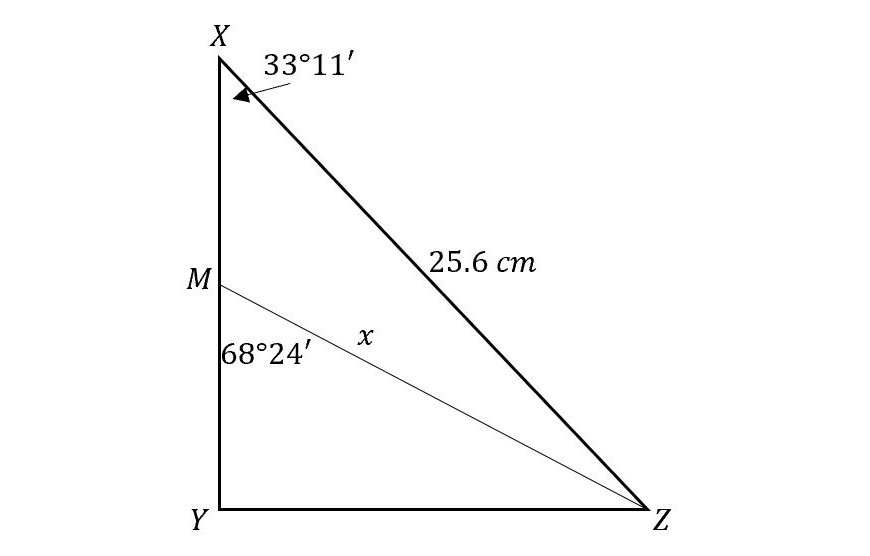3. The foot of a ladder makes an angle of $$60°$$ with the ground. The ladder is 20m long. How far is the ladder from the building?

4. From a window in Building $$A$$, the angle of elevation to the top of Building $$B$$ across the road measures $$36°$$. The angle of depression to the base of Building $$B$$ measures $$25°$$. The width of the road between the two buildings is 25m.

i) Draw a diagram representing the information given.

ii) Calculate the height of the Building $$B$$ correct to the nearest metre

iii) From the top of Building $$B$$, the angle of depression to the top of Building $$A$$ measures $$12°$$. Find the height of Building $$A$$, correct to the nearest metre.

5.

A ship $$A$$ is 250km due west of a lighthouse. It travels a distance of 120km north to $$C$$.

i) Draw a diagram to show the position of $$C$$.

ii) Calculate the distance from the lighthouse to $$C$$ to the nearest km.

iii) Find the bearing of $$C$$ from the lighthouse (as a true bearing, correct to the nearest minute)

## Solutions

1.

First, we draw out the two triangles and label in the information we have been given.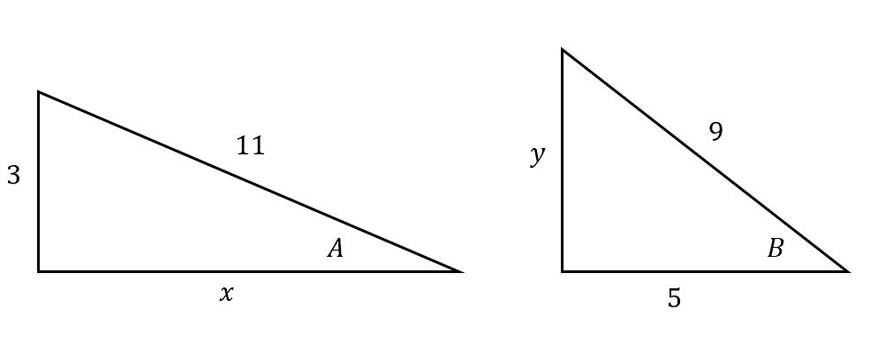Next, we must find the unknown values using Pythagoras’ Theorem.

$$11^2=3^2+x^2 \\ x^2=121-9=112 \\ x=10.6 \\$$

And

$$9^2=5^2+y^2 \\ y^2=81-25=56 \\ y= 7.5 \\$$

Now we can evaluate the trigonometric ratios.

$$cos⁡A=\frac{A}{H}=\frac{10.6}{11} \\ tan⁡B=\frac{O}{A}=\frac{7.5}{5} \\$$

2. To find $$YZ$$ we use the sine ratio as we are given an angle and the hypotenuse. Now we can evaluate the required trigonometric ratios.

$$sin⁡33°11’=\frac{YZ}{25.6} \\ YZ=24.6 \times sin⁡ 33°11’= 13.5cm \\$$

Next, in $$∆MYZ$$ we can use the sine ratio again to find $$x$$.

$$sin⁡68°24’=\frac{YZ}{x} \\ ∴x=\frac{YZ}{sin⁡68°24^{‘}} =14.5cm \\$$

3.

First, we need to sketch a diagram of the information given.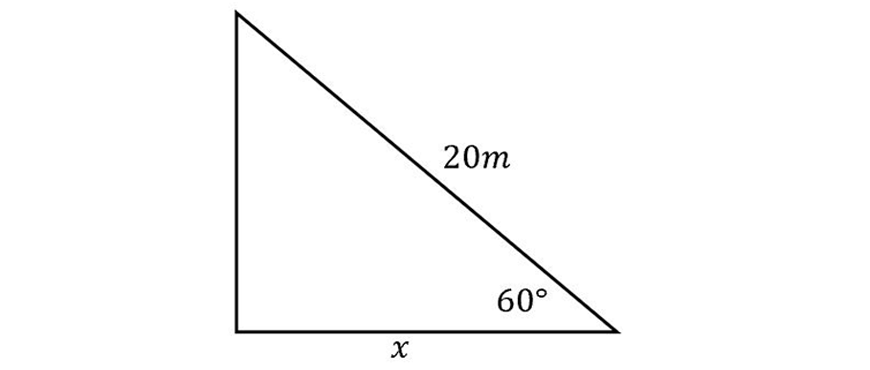The question is basically asking us to find $$x$$. For this we use the cosine ratio.

$$cos60°=\frac{x}{20} \\ x=20 cos⁡60° \\$$

We can use the exact value of $$cos60°$$.

$$x=20*\frac{1}{2} \\ ∴x=10m \\$$

4.

i) We must represent the information given to us: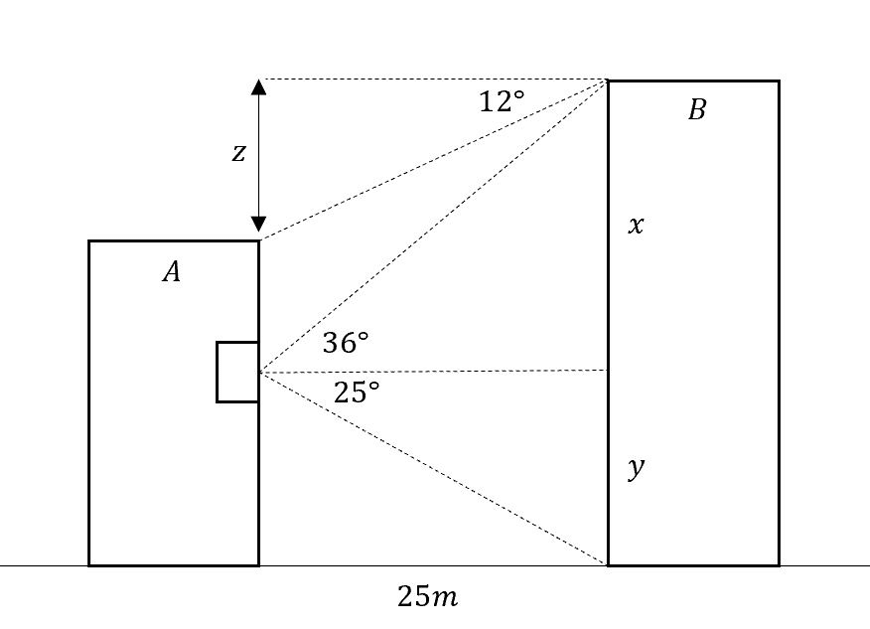ii) The height of Building $$B$$ is the sum of $$x$$ and $$y$$. We can find these individually and sum them together.

$$tan⁡36°=\frac{x}{25} \\ x=25 tan36°= 18.16m$$

And,

$$tan⁡25°=\frac{y}{25} \\ y=25 tan⁡25°= 11.66m \\ ∴ \text{height of B}=x+y= 30m$$

iii) To find the height of Building $$A$$, we can subtract the length $$z$$ from the height of Building $$B$$. To find $$z$$ , we use the tangent ratio.

$$tan⁡12°=\frac{z}{25} \\ z=25 tan⁡12°=5.31m \\ ∴\text{height of A}=30-5.31=24.69m \\$$

5)

i) Using the information given we draw a diagram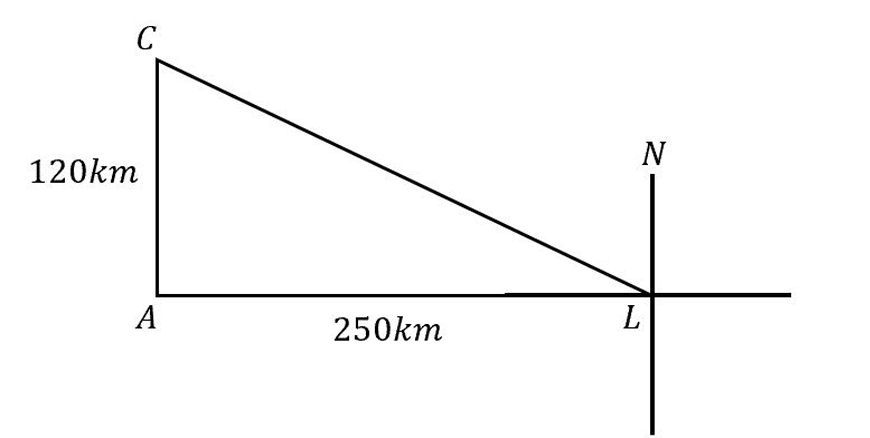ii) We are required to find the distance $$CL$$. This can be done using Pythagoras’ theorem.

$$CL^2=120^2+250^2 \\ CL^2=76900 \\ ∴CL=277.3085=277 km \\$$

iii) We first need to find $$\angle CLA$$ . This can be found using any two sides of the given right-angled triangle. We will use tangent ratio.

$$tan⁡θ=\frac{120}{250} \\ ∴ θ= tan^{-1}⁡\frac{12}{25}=25°38′ \\ ∴ \text{True bearing} =270°+ θ =295°38′ T \\$$

## Summary:

• The key to solving trigonometric questions is to correctly draw and interpret the right-angled triangle
• When asked to find an unknown side or angle, consider the information given for the right-angled triangle and which trigonometric ratio contains this information
• For exact solutions, consider the use of the exact value triangles. It is easiest to memorise and quickly draw out the two exact value triangles to find the exact value trigonometric ratios
• To construct the right-angled triangle, questions may refer to the angle of elevation and depression or use bearing. Carefully sketch out the information given and consider using properties of angles on parallel lines when solving.
• For questions using bearing we always start at the “from” point and go to the “to” point.
E.g. from O to P.

## Test out your Trigonometry skills!© Matrix Education and www.matrix.edu.au, 2021. Unauthorised use and/or duplication of this material without express and written permission from this site’s author and/or owner is strictly prohibited. Excerpts and links may be used, provided that full and clear credit is given to Matrix Education and www.matrix.edu.au with appropriate and specific direction to the original content.